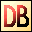DelphiBasics
 RandomizeProcedure Reposition the Random number generator next value System unit
 procedure Randomize();
Description
The Randomize procedure is used in conjunction with the Random function. It repositions the random number generator in its sequence of 232 pseudo random numbers.

Randomize uses the time of day as the seed for this repositioning, so should provide a reliable method of creating an unpredictable sequence of numbers, even if they are a part of a predetermined sequence.
Related commands
 Random Generate a random floating point or integer number RandomRange Generate a random integer number within a supplied range RandSeed Reposition the Random number generator next valueDownload this web site as a Windows program.

 Example code : Run this code twice to see the effect of Randomize var   int   : Integer;   i     : Integer; begin   // If you run this program twice, only the first 5 values   // will be guaranteed to be the same each time - randomize   // repositions into a different part of the pseudo sequence   // of random numbers.   // Get an integer random number in the range 1..100   ShowMessage('Fixed first 5 random numbers');   for i := 1 to 5 do   begin     int := 1 + Random(100);    // The 100 value gives a range 0..99     ShowMessage('int = '+IntToStr(int));   end;   // Now randomize to reposition   Randomize;   ShowMessage('');   // Get an integer random number in the range 1..100   ShowMessage('Random next 5 numbers');   for i := 1 to 5 do   begin     int := 1 + Random(100);    // The 100 value gives a range 0..99     ShowMessage('int = '+IntToStr(int));   end; end; Show full unit code Fixed first 5 random numbers   int = 1   int = 4   int = 87   int = 21   int = 28      Random next 5 numbers   int = 35   int = 74   int = 45   int = 50   int = 31